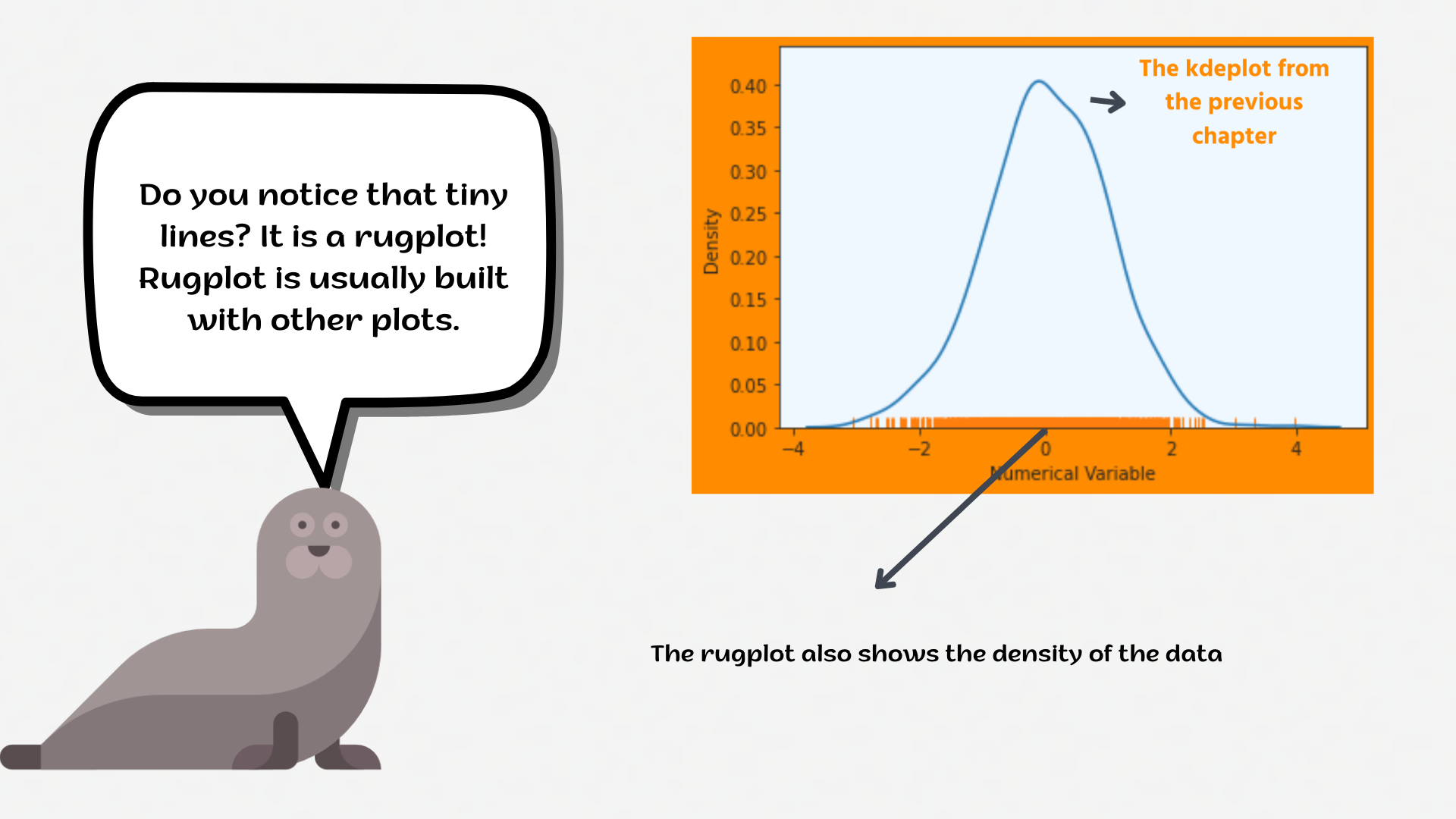Course Content

# Deep Dive into the seaborn Visualization

1. Light Start

2. Distributions of Data

3. Categorical Plot Types

4. Matrix Plots

5. Multi-Plot Grids

6. Regression Models

Deep Dive into the seaborn Visualization

##RugplotThe `rugplot` is intended to complement other plots by showing the location of individual observations in an unobtrusive way.1. Set the `'darkgrid'` style with the disabled `'axes.grid'` and the `'axes.facecolor'` equals the `'aliceblue'` (color). We learned how to do that in the first part of the course.
2. Create the `kdeplot` using the `seaborn` library.
3. Create the `rugplot` using the `seaborn` library:
• Set the `x` parameter equals the `'total_bill'`;
• Set the `hue` parameter equals the `'sex'`;
• Set the `height` parameter equals `0.05`;
• Set the `'magma'` `palette`;
• Set the data using the `df`;
• Display the plot.

Everything was clear?

Section 2. Chapter 3